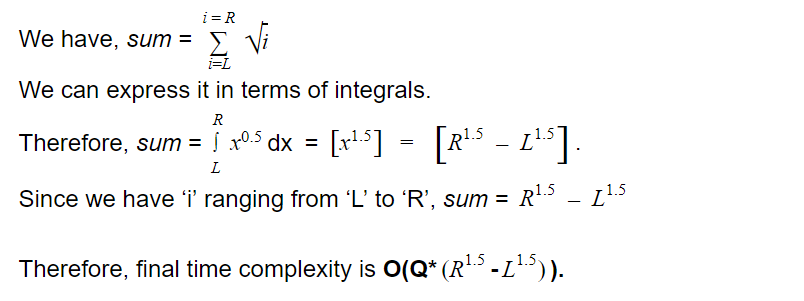# Count Prime in Ranges

## Introduction

Mohammed and Ali were two friends who lived in Medina. One day Ali asked Mohammed a difficult problem. But Mohammed is the master of everything. He asked Ali to present the question. Ali said that given two numbers ‘L’ and ‘R’, we need to find the count of all prime numbers between ‘L’ and ‘R’ inclusive. Mohammed said that it is easy to solve. He further said that we could iterate from ‘L’ to ‘R’ and check if it is prime or not. If it is a prime number, then we increment our count. Ali said that I thought of the same solution. Mohammed worked hard and then came up with the most optimal solution. Let us discuss these algorithms and their implementations.

## Problem Statement

Given two numbers, ‘L’ and ‘R’, and ‘Q’ queries, we need to find the count of all prime numbers between ‘L’ and ‘R’ inclusive for each query.

Given that 0 <= L <= R < 1000000, and 0 <= Q < 100000.

## Naive Algorithm

The brute force approach will be to iterate from ‘L’ to ‘R’ and for every number, check if the given number is prime or not. If it is a prime, then we increment the count. We will do the same for every query and print the count.

If you do not know how to check the primality of a number, refer to this blog to learn various concepts of checking the primality of a number. In this approach, we will use the sqrt(N) method to check whether a number is prime or not. With this said, let us now implement the brute force method.

### Time Complexity

O(Q *(R1.5-L1.5)), where ‘Q’ is the number of queries and L and R are the range endpoints.

The time complexity of the isPrime() function for an input ‘N’ is O(sqrt(N)). We are calling the isPrime() function for R - L + 1 times for different values of i. Thus the time complexity is O( Q *i = LRi) = .O(Q*(R1.5-L1.5)).

#### Proof:### Space Complexity

O(1).

As we are not using any auxiliary space.

## Optimized Approach

In this algorithm, we trade space with time. We need to lose at least one part. We can use the Sieve of Eratosthenes to find all the prime numbers between 1 and 1000000. Then we populate the prefix array. The value of the ‘i’th index of the prefix array gives the number of primes up till ‘i’. Finally, if we want to find the count of prime numbers between ‘L’ and ‘R’, we just calculate prefix[R]-prefix[L-1].

Let us implement this algorithm.

### Time Complexity:

For Precomputation: O(N*log(log(N))). (Here, we have taken N = 1000000).

For Query: O(1)

At first, we are calling the sieve() function to find all the primes <= 1000000. The generic sieve() implementation takes O(N * log(log(N))) time. After that, populating the prefix array takes another O(N) time. Thus, total precomputation cost is O(N * log (log(N)) + N) =  O(N * log (log(N))).

And to answer a query, it takes O(1) time.

### Space Complexity:

O(10 ^ 6).

The space complexity of the above algorithm is O(10 ^ 6) as we are using two arrays of size 10 ^ 6.

## Key Takeaways:

In this blog, we learned an exciting problem of number systems. This problem has been asked multiple times in coding interviews. You can practice similar problems and gain expertise in number systems on our well-crafted public platform, CodeStudio. We learned two ways to implement this problem. First, we saw a naive approach that took O(Q * (R1.5-L1.5)) time. Then we saw the implementation using Sieve of Eratosthenes, which took O(N*(log(log(N))) time for precomputation and O(1) for answering a query.  We hope you found this blog useful. To learn more, you can also check out our Competitive Programming course, which will make your coding journey easier and full of fun.